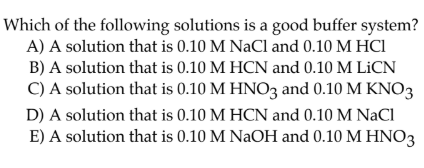# Which of the following solutions is a good buffer system? A) A solution that is 0.10 M NaCl and 0.10 M HCl B) A solution that is 0.10 M HCN and 0.10 M LiCN C) A solution that is 0.10 M HNO3 and 0.10 M KNO3 D) A solution that is 0.10 M HCN and 0.10 M NaCl E) A solution that is 0.10 M NaOH and 0.10 M HNO3